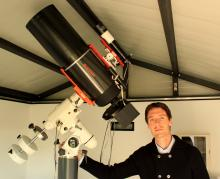# Proper use of the extinction coefficients

1 post / 0 new
r.furgoniProper use of the extinction coefficients

Hello,

i need to determine the correct B-V value for the star UCAC4 733-009103 (00 51 40.309 +56 30 33.38). The star is present in APASS with Bmag=13.648 and Vmag=13.337 so B-V is 0.311. The point is that I need to get the value corrected for the extinction at various wavelengths: I then entered the coordinates of the star in the NASA-IPAC extinction calculator but I'm not sure how to use the results.

Here the link to the page.

If for Landolt B the Aλ [mag] is 10.697 and for Landolt V the Aλ [mag] is 8.089, how the two values ​​must be used to obtain the correct B-V?

Thanks,

Riccardo (FRIC)